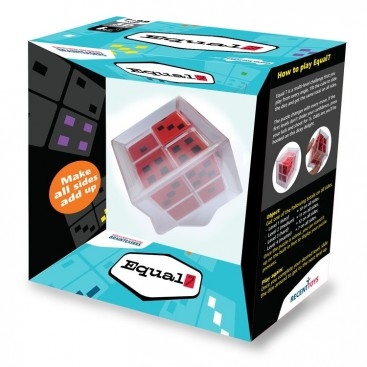Sale!View larger

Equal7

EQ7

New product

EQUAL7 is a perfect mathematical puzzle, with multiple activities and levels of difficulty.

9,95€ Tax included

-3,00€

Before 12,95€

• EQUAL7 is a perfect mathematical puzzle, with multiple activities and levels of difficulty. The levels are:

Level 1 (easy): slide the dice in such a way that all points on each side add up exactly 10.

Level 2 (medium): slide the dice in such a way that all points on each side add up exactly 11

Level 3 (difficult): slide the dice in such a way that all points on each side add up exactly 7, hence is name EQUAL7

Mode of play with EQUAL 7: pick up the cube, lean and move it. Then move the dice until you solve the challenge.